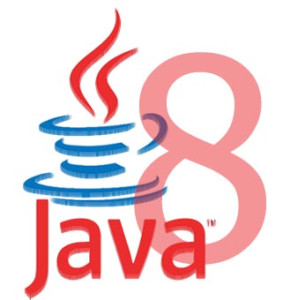Home Interview Questions and Answers Java 8 Interview Questions and Answers For Freshers Part-211.What is the purpose of DoublePredicate functional interface?
It represents a predicate (Boolean-valued function) of one double-valued argument.

12.What is the purpose of DoubleSupplier functional interface?
It represents a supplier of double-valued results.

13.What is the purpose of DoubleToIntFunction functional interface?
It represents a function that accepts a double-valued argument and produces an int-valued result.

14.What is the purpose of DoubleToLongFunction functional interface?
It represents a function that accepts a double-valued argument and produces a long-valued result.

15.What is the purpose of DoubleUnaryOperator functional interface?
It represents an operation on a single double-valued operand that produces a double-valued result.

16.What is the purpose of Function<T,R> functional interface?
It represents a function that accepts one argument and produces a result.

17.What is the purpose of IntBinaryOperator functional interface?
It represents an operation upon two int-valued operands and produces an int-valued result.

18.What is the purpose of IntConsumer functional interface?
It represents an operation that accepts a single int-valued argument and returns no result.

19.What is the purpose of IntFunction<R> functional interface?
It represents a function that accepts an int-valued argument and produces a result.

20.What is the purpose of IntPredicate functional interface?
It represents a predicate (Boolean-valued function) of one int-valued argument.

#### You may also like